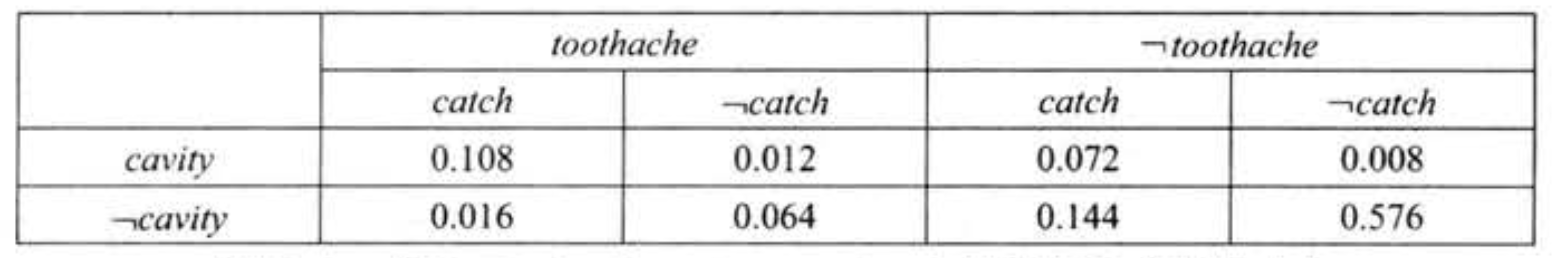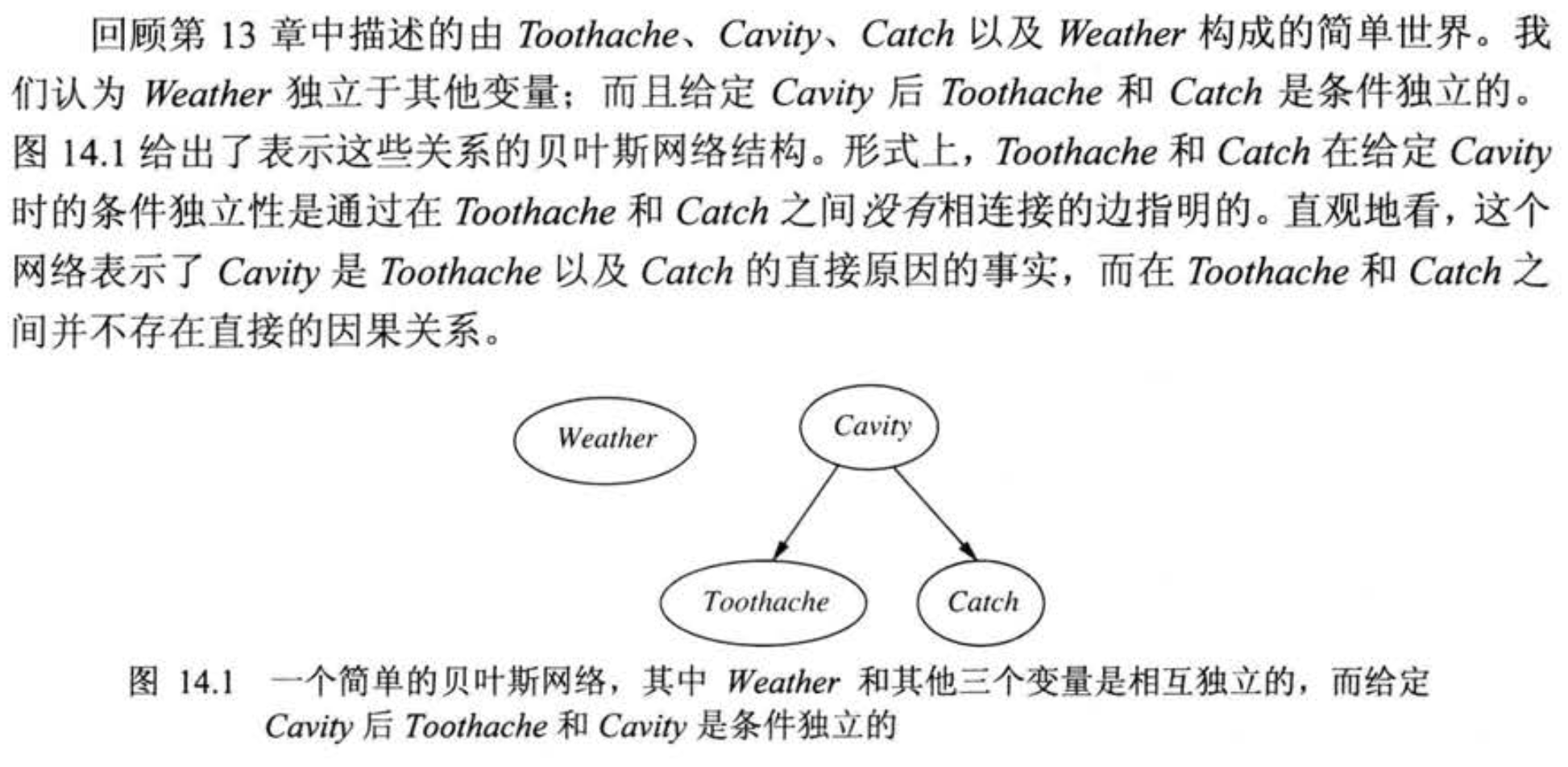# 人工智能导论 不确定性的量化和概率推理

## Uncertainty & Bayes Network

Posted by R1NG on December 20, 2021 Viewed Times

# 不确定性的量化和概率推理

## 4.1 不确定性

1. 要得到该问题的确定没有任何意外情况的规则需要列出前提和结论的完整集合, 而为了列出这个集合需要极为庞大的工作量, 给出的规则也难以使用.
2. 对于问题涉及的领域, 并不存在完整的结论能够支撑我们给出完备的规则.
3. 即便在给出了完备规则的前提下在一些情形下我们也无法进行完整的测试, 因此不能通过逻辑联系得出结论.

## 4.2 概率语法规则

$\text{for each }\omega, ~~0 \leqslant P(\omega) \leqslant 1, ~~ \sum_{\omega \in \Omega} P(\omega) = 1.$

$P(\text{isAxtonYao} ~ \vert ~\neg \text{isWeak} ~\wedge~ \text{isAxton}) = 0.9.$

$P( \text{Weather}, \text{Cavity}) = P( \text{Weather} ~\vert~ \text{Cavity}) \cdot P(\text{Cavity}).$

## 4.3 使用完全联合分布进行推理1. 首先, 最常见的任务是 提取关于随机变量的 某个子集某个变量 的概率分布, 这样的过程一般称为 边缘化 (Marginalization) 或 求和消元 (Summing Out).

对任何两个变量集合 $Y, Z$, 有下列的 通用边缘化规则:

$P(Y) = \sum_{z \in Z} P(Y, z)$

其中 $\sum_{z \in Z}$ 指针对变量集合 $Z$ 的所有可能取值组合进行求和. 我们还可以使用条件概率将上述规则变形如下:

$P(Y) = \sum_{z \in Z} P(Y \vert z) P(z).$
2. 在一些情况下, 我们还需要基于一些已知的变量证据而计算另一些变量的条件概率. 设 $E$ 为证据变量集合, $e$ 为其观察值, 并设 $Y$ 为其余的未观测变量, 查询为 $P(X \vert e)$, 则其值为:

$P(X \vert e) = \alpha P(X, e) = \alpha \cdot \sum_{y \in Y} P(X, e, y).$

其中 $\alpha = \frac{1}{P(e)}$, 对于 $\forall y \in Y$ 是不变的, 因此可以视为一个归一化常数.

## 4.4 应用贝叶斯规则

$P(b \vert a) = \frac{P(a \vert b) P(b)}{P(a)}.$

$P(Y \vert X) = \frac{P(X \vert Y) P(Y)}{P(X)}.$

## 4.5 不确定性问题域中的知识表示

1. 每个节点对应概率问题中的一个随机变量, 该变量既可以是离散的, 也可以是连续的.
2. 使用 一组有向边或箭头 连接节点对. 若有从 $X$ 指向 $Y$ 的箭头, 则称 $X$ 为 $Y$ 的一个父节点, 图中无圈 (也就是说这个图是一个有向无圈图, DAG).
3. 每个节点 $X_i$ 都有一个条件概率分布 $P(X_i ~\vert~ \text{Parents}(X_i))$ 用以 量化其父节点对该节点的影响.1. 将贝叶斯网络视为 对联合概率分布的表示.
2. 将贝叶斯网络视为 对一组条件依赖性语句的编码.

$P(X_1 = x_1 \wedge X_2 = x_2 \wedge \cdots \wedge X_n = x_n).$

$P(x_1, x_2, \cdots, x_n) = \prod_{i=1}^{n} \theta(x_i ~ \vert ~ \text{parents}(X_i))$

$P(x_1, x_2, \cdots, x_n) = \prod_{i=1}^{n} P(x_i ~ \vert ~ \text{parents}(X_i)).$

\begin{aligned}P(x_1, x_2, \cdots, x_n) &= P(x_n ~\vert~ x_{n-1}, \cdots, x_1) \cdot P(x_{n-1} ~\vert~ x_{n-2}, \cdots, x_1) \cdots P(x_2 ~\vert~ x_1)\cdot P(x_1) \\ &=\prod_{i=1}^{n} P(x_i ~\vert~ x_{i-1}, \cdots, x_1). \end{aligned}

$P(X_i ~\vert~ X_{i-1}, \cdots, X_1) = P(X_i ~\vert~ \text{Parents}(X_i)).$

1. 给定节点 (Nodes): 确定对 问题域建模所需的变量集合, 对变量排序得一个序列 ${X_1, \cdots, X_n}$. 注意, 若在排序时让原因排列在结果前, 则我们得到的网络会更致密.
2. 给定边: 从 $1$ 到 $n$ 遍历变量 $i$, 对每个 $i$, 执行:

2.1 从 $X_1, \cdots, X_{i-1}$ 中选择 $X_i$ 的父节点的最小集合使得

$P(X_i ~\vert~ X_{i-1}, \cdots, X_1) = P(X_i ~\vert~ \text{Parents}(X_i)).$

得到满足.

2.2 在每个父节点和 $X_i$ 之间插入一条边.

2.3 写出条件概率表 $P(X_i ~\vert~ \text{Parents}(X_i)).$

## 4.6 贝叶斯网络的精确推理

1. 查询变量用 $X$ 表示.
2. 证据变量集 $E_1, \cdots, E_m$ 用 $E$ 表示.
3. 观察到的特定事件用 $e$ 表示.
4. 非证据, 非查询变量 (隐藏变量) 集 $Y_1, \cdots, Y_l$ 用 $Y$ 表示.

$X = \{X\} \cup E \cup Y.$

$P(X \vert e) = \alpha P(X, e) = \alpha \cdot \sum_{y \in Y} P(X, e, y).$